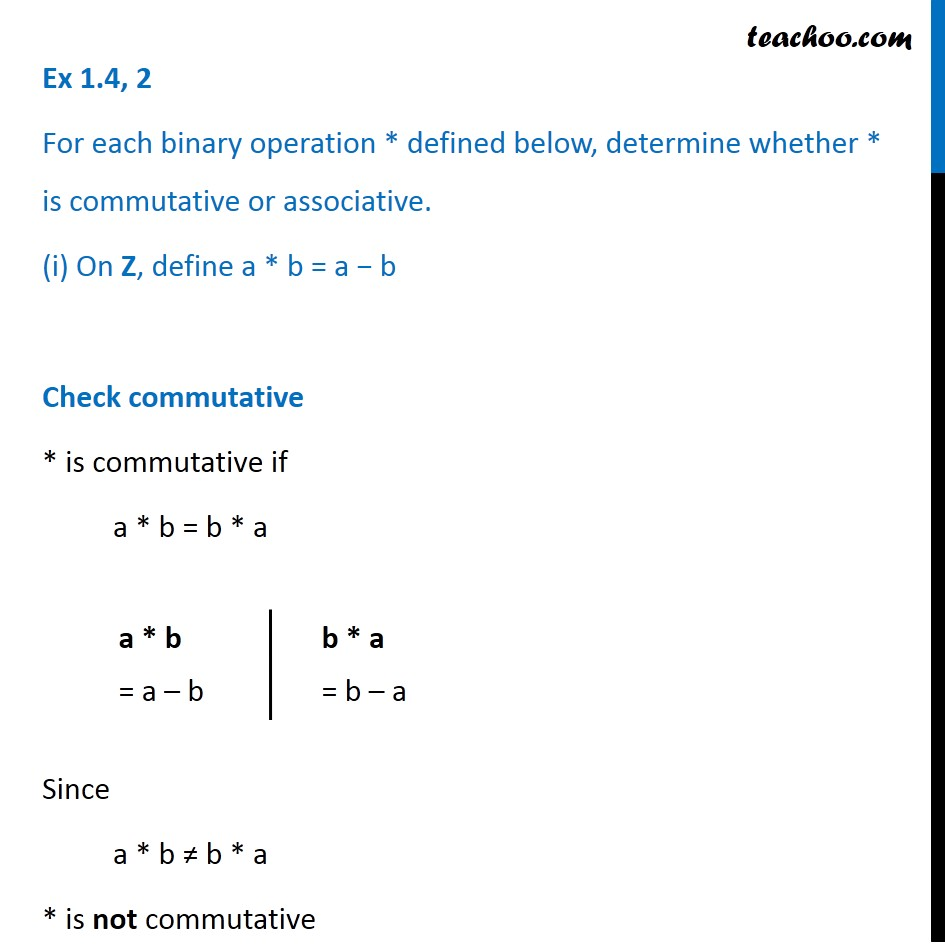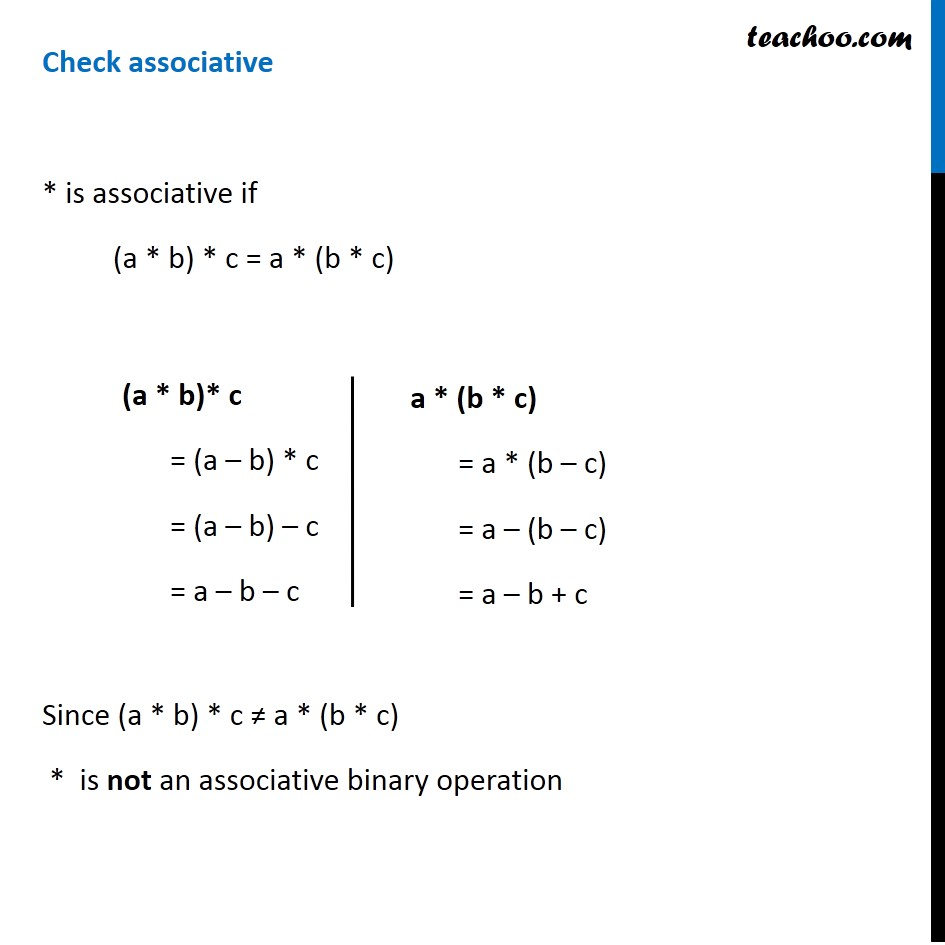Whether binary commutative/associative or not

Chapter 1 Class 12 Relation and Functions
Concept wiseLearn in your speed, with individual attention - Teachoo Maths 1-on-1 Class

### Transcript

Ex 1.4, 2 For each binary operation * defined below, determine whether * is commutative or associative. (i) On Z, define a * b = a − b Check commutative * is commutative if a * b = b * a Since a * b ≠ b * a * is not commutative a * b = a – b b * a = b – a Check associative * is associative if (a * b) * c = a * (b * c) Since (a * b) * c ≠ a * (b * c) * is not an associative binary operation (a * b)* c = (a – b) * c = (a – b) – c = a – b – c a * (b * c) = a * (b – c) = a – (b – c) = a – b + c